# Stochastic processes, filtering of

filtration of stochastic processes

The problem of estimating the value of a stochastic process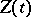at the current momentgiven the past of another stochastic process related to it. For example, estimate a stationary processgiven the values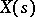,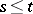, of a stationary process stationarily related to it (see , for example). Usually one considers the estimatorwhich minimizes the mean-square error,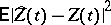. The use of the term "filter" goes back to the problem of isolating a signal from a "mixture" of a signal and a random noise. An important case of this is the problem of optimal filtering, when the connection betweenand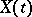is described by a stochastic differential equation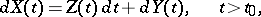where the noise is assumed to be independent ofand is given by a standard Wiener process.

A widely used filtering method is the Kalman–Bucy method, which applies to processesthat are described by linear stochastic differential equations. For example, if, in the above scheme,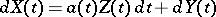with zero initial conditions, then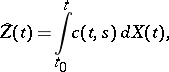where the weight functionis obtained from the equations: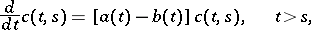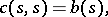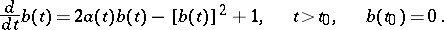The generalization of this method to non-linear equations is called the general stochastic filtering problem or the non-linear filtering problem (see ).

In the case when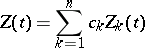depends on the unknown parameters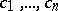, one can obtain the interpolation estimator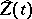by estimating these parameters given,; the method of least squares applies here, along with its generalizations (see , for example).